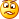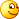Guest post by David Churchward [Twitter]Firstly, I have to be clear that I’m not presenting a “one-size-fits-all” approach to sales commission calculations here.  That wouldn’t be possible because commission schemes vary extensively and, in my experience, some schemes aren’t even based on a logic that can derive a mathematical answer!  However, I’m presenting an approach here that will hopefully provide a template approach that can be modified to a number of different scenarios.

### The Target Outcome

The scheme that I’m using in this example operates as follows:

1. Commission is paid monthly based on the achievement in that month
2. As a salesperson sells more, then accelerators trigger.  That is to say, for example, a salesperson may receive 1% of sales up to £10,000 and 2% of sales between £10,000 and £20,000.  The 2% is payable on the whole value.  Therefore, if a salesperson sells £11,000 then they would receive 2% of the full £11,000
3. Percentages and bands can change monthly at management discretion
4. This is a monthly threshold so each salesperson is reset to zero at the start of each month.
5. Each product group carries a separate set of bands and rates
6. Managers receive commission at a different rate to the sales team based on the total sales for their team.

Naturally, this is a hypothetical dataset and commission scheme but it is closely based on a real life example that I implemented recently.  I’ve hacked together a dataset as I couldn’t release my real life example for obvious reasons.

### The Dataset

Before I go into the schema, it’s worthwhile noting once again that I have hacked this dataset together.  Because I lack the imagination to dream up names for members of the sales team and also because I was just reading a write up on Liverpool’s drubbing of Chelsea yesterday, I’ve used the Liverpool squad reporting into “King Kenny”.  Obviously, I’m not implying for one minute that my beloved Liverpool team should turn their hand to selling AV and Computer electricals!Note – I’ve compiled this workbook in PowerPivot V1 but quickly whipped it through V2 to get the nice table view above!I have four related tables

1. Orders– this is a list of orders detailing the usual candidates for a dataset like this – Order (being the order number), Date, Salesman, Qty, unit_Cost, Value and Type.
2. People– this is a list of the unique salesperson names and the manager to whom they report.
3. Types– this is a product grouping.  As my commission scheme carries different parameters and rates dependent on the product group, I have to be able to analyse my dataset at this level.  In this case, I have two product groups being AV (Audio Visual) and COMP (Computers)
4. Dates– this is a fairly standard dates table.  I have a sequential list of dates with a number of attributes.  In truth, most of these attributes are redundant for this analysis.  The main attribute is the MonthEndDate which I will use in the DAX measure that we create.
5. Rates – this is NOT RELATED to any other tables.  The reason for this is because I want to call in information in different sections of this table that, in turn, is dependent on the outcome of a dynamic measure.

### The Dax

#### A Simple “Starter for 10”

Obviously, we need to calculate how much has been sold by an individual salesperson in a given month in order to then calculate how much commission accrues as a result.  My final analysis is going to be carrying People[Name], People[Manager] and Dates[MonthEndDate] on rows in my report.  As a result, I don’t have to worry about applying any cunning filters.  The upshot is that we can resort to a simple SUM() measure.

Sales_Value

=SUM(Orders[Value])

Rob – is there a level 0 on the spicy scale?

#### And then it gets a “bit” harder”!

Whilst Sales_Value may not be the most intricate DAX expression you’ve ever seen, things get a bit more tasty when we try to determine which percentage rate to apply in our calculation.  I’ll dive straight into the DAX.

Comm_Rate

=IF(COUNTROWS(VALUES(Dates[MonthEndDate]))=1

&&COUNTROWS(VALUES(People[Name]))=1

&&COUNTROWS(VALUES(Types[Type_Code]))=1,

IF([Sales_Value]=BLANK(),BLANK(),

CALCULATE(MAX(Rates[Rate]),

FILTER(Rates,

Rates[From]<=[Sales_Value]

&&Rates[To]>=[Sales_Value]

&&Rates[From_Date]<=MAX(Dates[MonthEndDate])

&&Rates[To_Date]>=MAX(Dates[MonthEndDate])

&&Rates[Type]=VALUES(Types[Type_Code])

&&Rates[Rate_Group]=”Salesperson”

)

)

),

IF(COUNTROWS(VALUES(Dates[MonthEndDate]))=1

&&COUNTROWS(VALUES(People[Name]))>1

&&COUNTROWS(VALUES(Types[Type_Code]))=1,

IF([Sales_Value]=BLANK(),BLANK(),

CALCULATE(MAX(Rates[Rate]),

FILTER(Rates,

Rates[From]<=[Sales_Value]

&&Rates[To]>=[Sales_Value]

&&Rates[From_Date]<=MAX(Dates[MonthEndDate])

&&Rates[To_Date]>=MAX(Dates[MonthEndDate])

&&Rates[Type]=VALUES(Types[Type_Code])

&&Rates[Rate_Group]=”Manager”

)

)

)

)

)

Let’s break that down.

We only want to evaluate a rate when:

1. We have one MonthEndDate by using IF(COUNTROWS(VALUES(Dates[MonthEndDate]))=1. It doesn’t make sense to calculate at any other point through the aggregation.  If we were to do so, the fact that we’re using MAX(Rates[Rate]) would mean that the highest value over a combination of months would be returned at an aggregation level where multiple months were included.
2. We want to calculate a rate for each salesperson independently.  To do this, we add a further condition &&COUNTROWS(VALUES(People[Name]))=1.
3. We have different rates for each Type (where Type is the product group – in this case Audio Visual and Computers)
4. We only want to return a value when we have a Sales_Value.  This is more to do with aesthetics than anything else.  If you have a Salesperson who doesn’t sell anything or wasn’t perhaps employed at a point in time of the analysis, you’ll get a series of unnecessary additional lines.  We therefore exclude those situations using IF([Sales_Value]=BLANK(),BLANK().

Where these conditions hold true, we want to pick the appropriate rate.

#### The Rates Table

Before we get onto the FILTER() expression, it probably makes sense to take a good look at the Rates table to be clear on what we’re looking at:Remember that this table ISN’T related to any of our other tables.  As a result, we have to provide all of the conditions that will filter this list down to one result and we can then return the Rates[Rate] value.  As a result, we need to plot the conditions below within a FILTER() condition:

1. Our [Sales_Value] must fit between the Rates[From] and Rates[To] values.  You’ll notice that the values in this rates table follow on from one another so we should be able to guarantee a result.  This is done using Rates[From]<=[Sales_Value]&&Rates[To]>=[Sales_Value]
2. We need to provide a value for Rates[Type] as we could conceivably return multiple results for multiple products and our measure will therefore have to evaluate a MAX() result.  This is done using &&Rates[Type]=VALUES(Types[Type_Code])
3. We can return different rates for different periods in time.  The Order can be pigeon holed between Rates[From_Date] and Rates[To_Date] using &&Rates[From_Date]<=MAX(Dates[MonthEndDate])&&Rates[To_Date]>=MAX(Dates[MonthEndDate])
4. Finally, because we can get a different rate for a Salesperson from a Manager, we need to explicitly state that &&Rates[Rate_Group]=”Salesperson”.  You’ll recall that our upfront IF() statement has already determined that we’re evaluating at the Salesperson level using &&COUNTROWS(VALUES(People[Name]))=1

Having done all of that, we then do the same again but for Managers.  The only difference is that we evaluate when &&COUNTROWS(VALUES(People[Name]))>1  to determine that we’re at the manager level (based on a Manager having more than one Salesperson reporting to them) and &&Rates[Rate_Group]=”Manager” for obvious reasons.

The outcome with these measures in place shows the following:### So what next?

Surely, we can now calculate commission using [Sales_Value]*[Comm_Rate] right?  If it were that easy, I wouldn’t be lining up a parting comment to say that I’ll be back with a follow up to show how it’s done.  To whet appetites, think nested SUMX!0 Shares